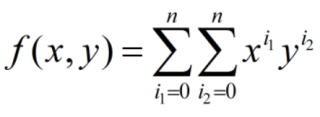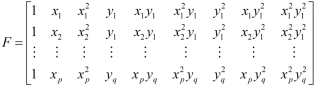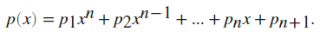# MATLAB如何实现带等式和不等式约束条件的最小二乘法拟合多元多项式``````Z=xlsread('Linton.xlsx');
x=Z(:,1);%x的数据，为风速，范围是0到40
y=Z(:,2);%y的数据，为风向，范围是0到360
z=Z(:,3);%频率数据
n = 7; % n为多项式的阶数，参数一共为(n+1)²个
p = length(x); q = length(y);
[X,Y,i1,i2] = ndgrid(x,y,0:n,0:n);
F = X.^i1.*Y.^i2;
F = reshape(F, p*q, (n+1)^2); %这一段是最小二乘法的左边矩阵，F矩阵具体形式如下图
A = F'*F;
b = F'*z;
a = A\b;%最小二乘法
````````````G = 1./(n+1:-1:1).*40.^(n+1:-1:1);% 这段是上图的普通多项式积分为1，直接写出了积分形式然后用拉格朗日乘子法
%现在需要对最上面的多元多项式积分为1，需要改成x在0到40，y在0到360的二重积分为1
aa = [A, F'; F, 0]\[b;1];%拉格朗日乘子法
``````

• 写回答

#### 悬赏问题

• ¥15 苹果手机删除了qq软件，qq文件怎么恢复
• ¥20 用mips 人力编程排序问题（能在mars按要求运行）
• ¥15 Verilog小型神经网络(单层反馈神经网络)
• ¥15 设计一个数字钟，实现时分秒的显示
• ¥15 Clion使用头文件链接两个cpp
• ¥15 51单片机无法触发外部中断问题
• ¥15 基于半监督，无监督的调制识别
• ¥15 cst求 阵列 rcs 时候 频域 时域求解器 应该怎样设置 才让两者 计算结果 相似 请附图
• ¥15 关于#51单片机#的问题：大家帮我看一下为什么我的计时器数字一直不稳定
• ¥15 Qt 在应用程序里总是有[PREVIEW] recv window msg, name:(Qt5QWindowIcon) hwd:(287097a)等信息不断输出，怎么解决